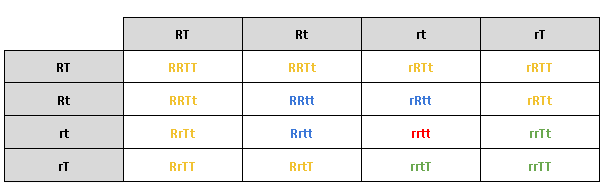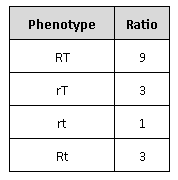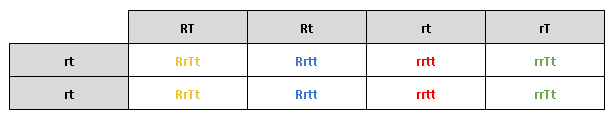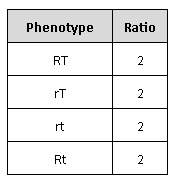# IMAT 2016 Q37 [Phenotypes]

An experiment is set up to study two genes. The two genes assort independently and for each gene the expression of alleles involves complete dominance. In the genetic cross RrTt x RrTt, a number of different phenotypes are seen. What is the difference between this number of phenotypes and the number of phenotypes seen when the cross RrTt x rrtt is carried out?

A. 4
B. 8
C. 2
D. 1
E. 0

It is said that the 2 genes have 2 different alleles :

• R and r
• T and t

Let’s now arbitrarily decide that R and T are the dominant alleles.

Genetic cross between RrTt x RrTt

A genetic cross between RrTt x RrTt gives us the following table :From this, we can determine that there are 4 different phenotypes that can result from the genetic cross between RrTt x RrTt.

They come in different ratios :Genetic cross between RrTt x rrtt

Similarly, we can do the following table :There are less genetic combinations but the same 4 phenotypes :Therefore, the difference between this number of phenotypes in the first cross and the number of phenotypes seen when the cross RrTt x rrtt is carried out is 0.